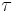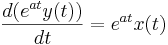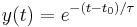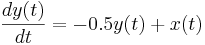# 7.2: First-order differential equations

•• Contributed by Peter Woolf et al.
• Assistant Professor (Chemical Engineering) at University of Michigan

## 2.1 Introduction

We consider the general first-order differential equation:

$\tau \frac{d y(t)}{d t}+y(t)=x(t)$

The general solution is given by:

$y(t)=y_{0} e^{-\left(t-t_{0}\right) / \tau}+\frac{e^{-\left(t-t_{0}\right) / \tau}}{\tau} \int_{t_{0}}^{t} x\left(t^{\prime}\right) e^{\left(t^{\prime}-t_{0}\right) / \tau} d t^{\prime}$

where y0 = y(t = t0). Note that t' is used to be distinguished from the upper limit t of the integral.

To obtain the general solution, begin with the first order differential equation:

$\tau \frac{d y(t)}{d t}+y(t)=x(t)$

Divide both sides by:

$\frac{d y(t)}{d t}+\frac{1}{\tau} y(t)=\frac{1}{\tau} x(t)$

Rewrite the LHS in condensed form using the integrating factor et / τ:Notice how a chain differentiation will return the LHS to the previous form

Simplify:

$\frac{d}{d t}\left[e^{t / \tau} y(t)\right]=\frac{1}{\tau} x(t) e^{t / \tau}$

Integrate both sides:

$e^{t / \tau} y(t)-e^{t_{0} / \tau} y\left(t_{0}\right)=\frac{1}{\tau} \int_{t_{0}}^{t} x\left(t^{\prime}\right) e^{t^{\prime} / \tau} d t^{\prime}$

Solve for y(t):

$y(t)=y_{0} e^{-\left(t-t_{0}\right) / \tau}+\frac{e^{-t / \tau}}{\tau} \int_{t_{0}}^{t} x\left(t^{\prime}\right) e^{t^{\prime} / \tau} d t^{\prime}$

## 2.2 Example Solutions of First Order Differential Equations

Consider:Multiplying both sides by dt gives:The general solution is given as:Now Consider:Dividing both sides by y(t) gives:which can be rewritten as:Multiplying both sides by dt, integrating, and setting both sides of the equation as exponents to the exponential function gives the general solution:Now Consider:The detailed solution steps are as follows:

1. Separate y(t) and x(t) terms2. Rewrite the LHS in condensed form using the "integrating factor" eatNotice how a chain differentiation will return the LHS to the form written in step 1

3. Divide both sides by eat4. Multiply both sides by dt and integrateThe general solution is given as:## 2.3 Step Function Simplification

$\tau \frac{d y(t)}{d t}+y(t)=x(t)$

For, which is the step function andThat is,forandotherwise:

The previously derived general solution:

$y(t)=y_{0} e^{-\left(t-t_{0}\right) / \tau}+\frac{e^{-\left(t-t_{0}\right) / \tau}}{\tau} \int_{t_{0}}^{t} x\left(t^{\prime}\right) e^{\left(t^{\prime}-t_{0}\right) / \tau} d t^{\prime}$

reduces to:, since the integral equals 1.

The quantitycan be seen to be the time constant wherebydrops toof its original value

## 2.4 Sample Problem

Problem Statement:

Consider the differential equationwhereAssuming, what is the behavior of?

Solution

General solution was derived previously as:

$y(t)=y_{0} e^{-a\left(t-t_{0}\right)}+e^{-a t} \int_{t_{0}}^{t} x\left(t^{\prime}\right) e^{a\left(t^{\prime}-t_{0}\right)} d t^{\prime}$

Forand, while setting, the solution reduces to:

$y(t)=e^{-0.5 t} \int_{0}^{t}\left(2+0.01 t^{\prime}\right) e^{0.5\left(t^{\prime}\right)} d t^{\prime}$

The following link may be referred to for integral tables: S.O.S. Math

Simplifying the solution gives the following:

$y(t)=\frac{\left.\left(e^{-0.5 t}\right)\left((x+198)\left(e^{0.5 t}\right)-198\right)\right)}{50}$

Plotting this function displays the following behavior:As can be seen clearly from the graph, initially the systemic response shows more exponential characteristics. However, as time progresses, linear behavior dominates.